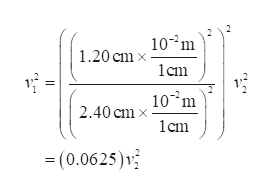Question
2 views

The Venturi tube shown in Figure P9.48 may be used as a fluid flowmeter. Suppose the device is used at a service station to measure the flow rate of gasoline (ρ = 7.00 x 102 kg/m3 ) through a hose having an outlet radius of 1.20 cm. If the difference in pressure is measured to be P1 - P2 = 1.20 kPa and the radius of the inlet tube to the meter is 2.40 cm, find (a) the speed of the gasoline as it leaves the hose and (b) the fluid flow rate in cubic meters per second.

check_circle

Step 1

(a) Using the equation of continuity,

Step 2

Substitute the values in equation (1) to find the relation between the velocities.help_outlineImage Transcriptionclose10-°m | 1.20 cm x 1cm v = 10°m 2.40 cm x 1cm = (0.0625)v || fullscreen
Step 3

Applying Bernoulli’s th...

### Want to see the full answer?

See Solution

#### Want to see this answer and more?

Solutions are written by subject experts who are available 24/7. Questions are typically answered within 1 hour.*

See Solution
*Response times may vary by subject and question.
Tagged in

### Physics